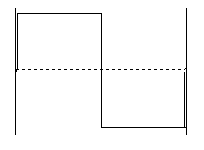TUTORIAL QUESTIONS 230-235

230.  A standing complex wave on a string of length 4 ft is made by adding the fundamental, f0, and a fourth harmonic of frequency 4 Hz.  What is the repeat time of this standing complex wave?

Fundamentals of Sound reference:Secs. 7-B, 7-D.

232. What is the symmetry of the complex wave shown on this string tied at both ends?a) symmetric
b) antisymmetric
c) mixed symmetry

Fundamentals of Sound reference:Sec. 7-B.

233. What is the repeat time of the complex wave shown in Question 232?

a) The period of the fundamental.
b) The period of the second harmonic.
c) The average of the periods of all the harmonics used in making the wave.
d) One can't tell from the information given.

Fundamentals of Sound reference:Secs. 7-B, 7-D.

235.  Three complex sound waves are created by adding different frequencies in the harmonic series based on f0 = 10 Hz, that is 10 Hz, 20 Hz, 30 Hz, 40 Hz, etc.  The three waves contain the following constituent frequencies:

Wave 1: 10 Hz and 20 Hz
Wave 2: 10 Hz, 20 Hz, and 40 Hz.
Wave 3: 10 Hz, 50 Hz, and 100 Hz.

a) The repeat time of all 3 waves is the same
b) The repeat time of all three waves is 1/10 s
c) All three waves may have different  repeat times
d) The repeat time of the 3 waves depends on the phases of the constituent frequencies,  so we can't tell anything about the repeat time
e) Both a) and b)
f) Both c) and d)
g) None of the above

Fundamentals of Sound reference:Secs. 7-B, 7-D.

Back to Tutorial Listing